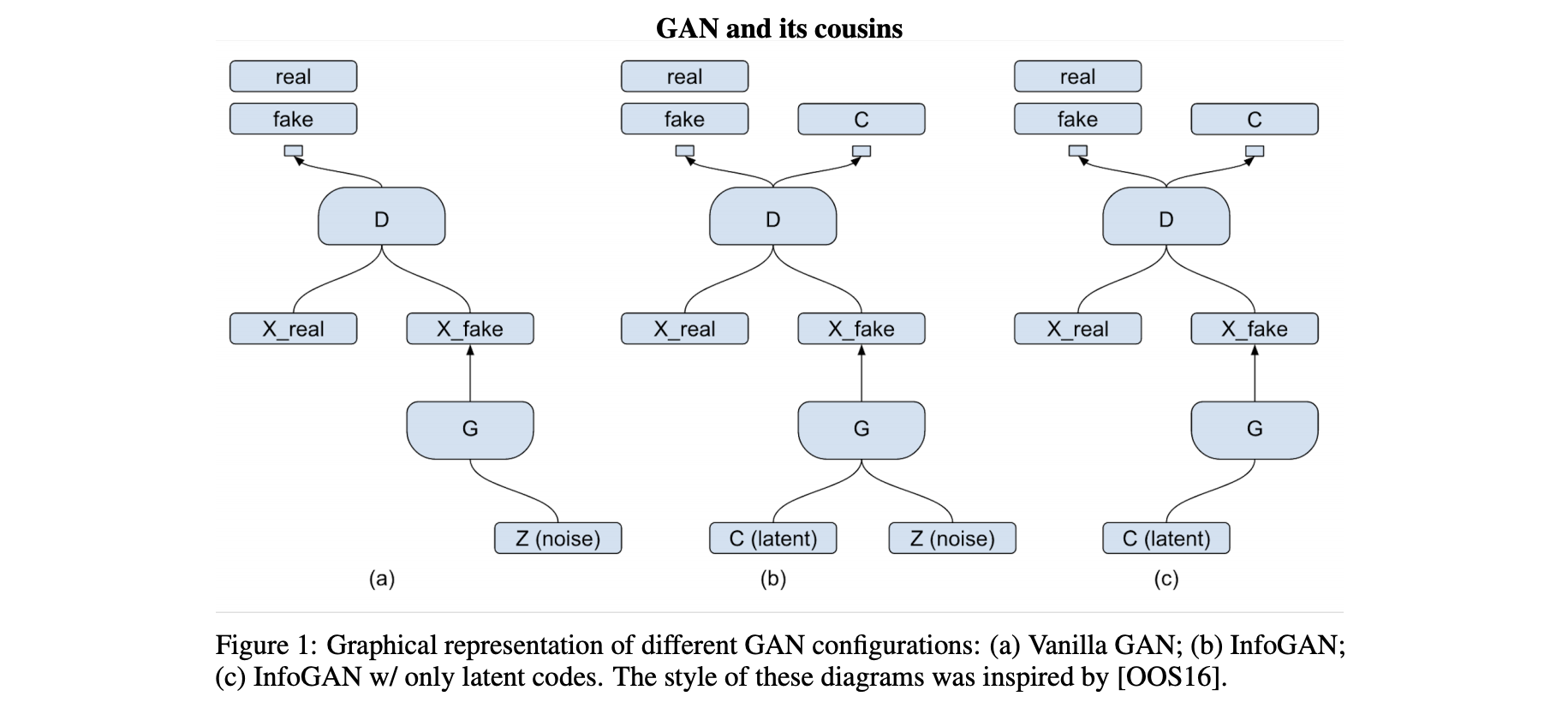N1H111SM's Miniverse

# InfoGAN

2020/06/08 ShareMaterials

# Motivation

Problem of unsupervised representation learning. While unsupervised learning is ill-posed because the relevant downstream tasks are unknown at training time, a disentangled representation, one which explicitly represents the salient attributes of a data instance, should be helpful for the relevant but unknown tasks. Thus, to be useful, an unsupervised learning algorithm must in effect correctly guess the likely set of downstream classification tasks without being directly exposed to them.

How to learn disentangled representation in this paper. In this paper, we present a simple modification to the generative adversarial network objective that encourages it to learn interpretable and meaningful representations. We do so by maximizing the mutual information between a fixed small subset of the GAN’s noise variables and the observations, which turns out to be relatively straightforward.

# Methods

## Mutual Information for Inducing Latent Codes

In this paper, rather than using a single unstructured noise vector, we propose to decompose the input noise vector into two parts: (i) $z$, which is treated as source of incompressible noise; (ii) $c$, which we will call the latent code and will target the salient structured semantic features of the data distribution.

We provide the generator network with both the incompressible noise z and the latent code c, so the form of the generator becomes $G(z, c)$. However, in standard GAN, the generator is free to ignore the additional latent code $c$ by finding a solution satisfying $P_G(x|c) = P_G(x)$. 为了解决trivial codes的问题，我们认为code $c$ 和生成结果generator distribution $G(z, c)$之间有较强的dependency，也就是说 $I(c; G(z,c))​$ 应当较高. 最终，InfoGAN优化的目标为以下：

## Variational Mutual Information Maximization

PASS. We will use InfoMax method to reproduce the experimental results.# Experiments

GAN的实验部分通常都比较偏经验性，并没有太好的Numerical Metrics来进行比较。值得一提的是，InfoGAN和之前看到的一篇未投稿工作一样，在MNIST数据集上验证时将latent code设置成了$K=10$ 的one-hot coding。我认为在这种setting下，representation进入下游分类任务取得很好的成绩是因为10-way classification是关于下游任务非常重要的信息泄露。